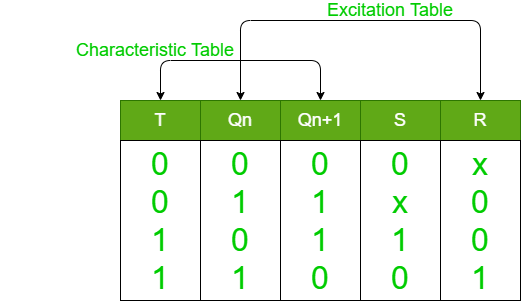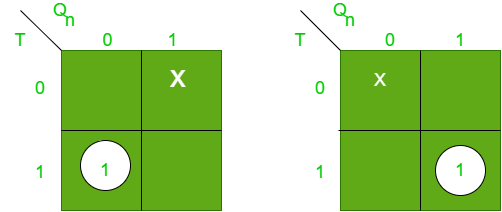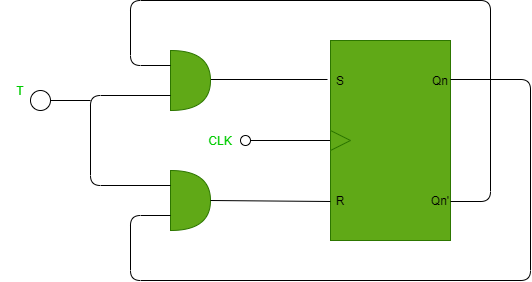Related Articles

# Conversion of S-R Flip-Flop into T Flip-Flop

• Last Updated : 22 Apr, 2020

Prerequisite – Flip-flop
Here, we will discuss the process of conversion of S-R Flip-Flop into a T Flip-Flop using an example.

Rules for conversion:

• Step-1:
Find the characteristics table of required flip-flop and the excitation table of the existing (given) flip-flop.
• Step-2:
Find the expression of given flip-flop in terms of required flip-flop using K-map.
• Step-3:
Find the circuit diagram of required flip-flop.

S-R Flip-Flop to T Flip-Flop:
Following is the characteristics table of T flip-flop and excitation table of S-R flip-flop.Now, using K-map we get the expression for of S & R in terms of T.S = TQn’ & R = TQn

Therefore, the circuit diagram for conversion of S-R flip-flop into T flip-flop is:Attention reader! Don’t stop learning now.  Practice GATE exam well before the actual exam with the subject-wise and overall quizzes available in GATE Test Series Course.

Learn all GATE CS concepts with Free Live Classes on our youtube channel.

My Personal Notes arrow_drop_up